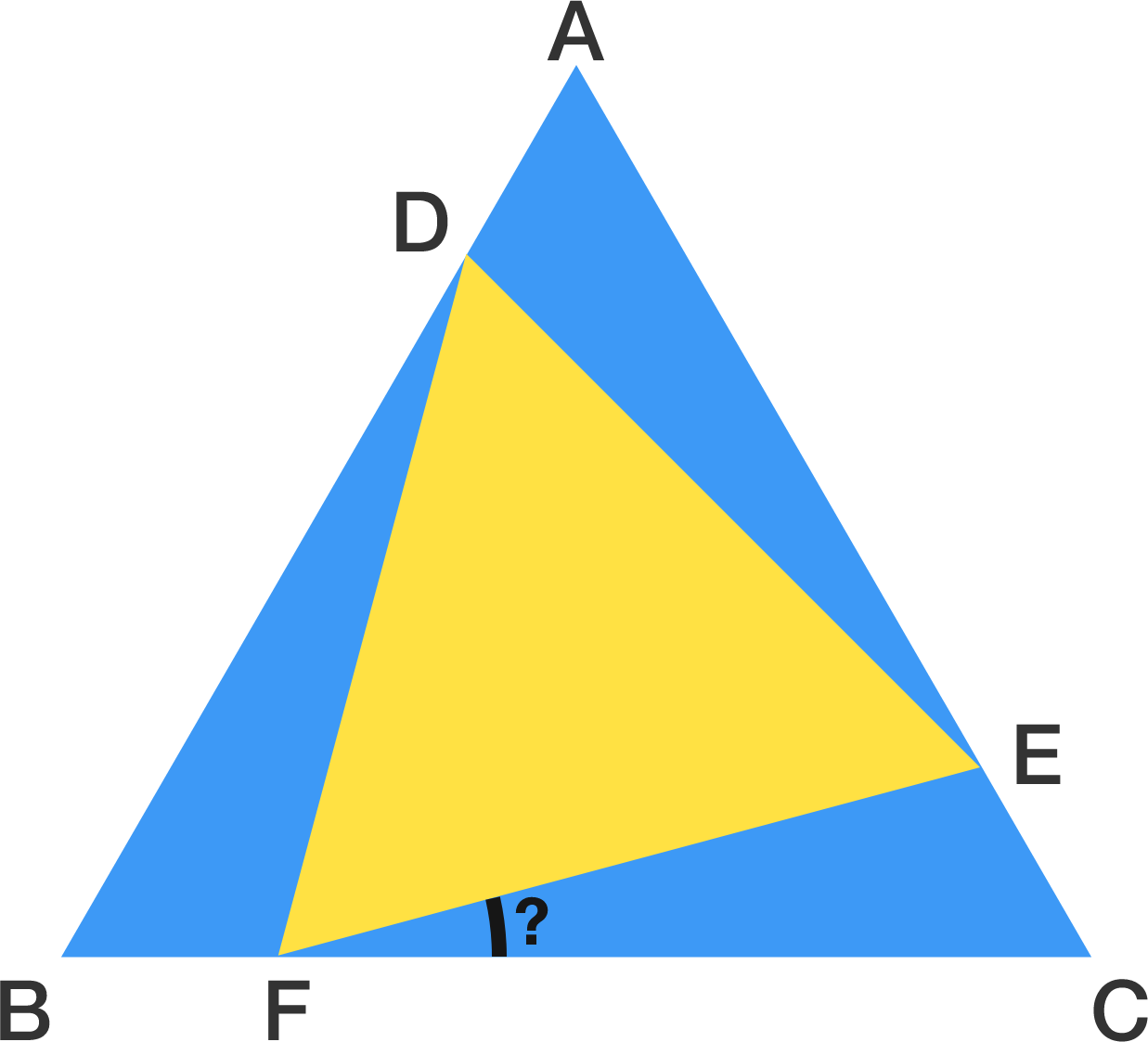# Triangles Being Fair

Geometry Level 2Triangles $ACB$ and $DEF$ are both equilateral, and the blue area is equal to the yellow area. Find the acute angle $\angle EFC$ in degrees.

×